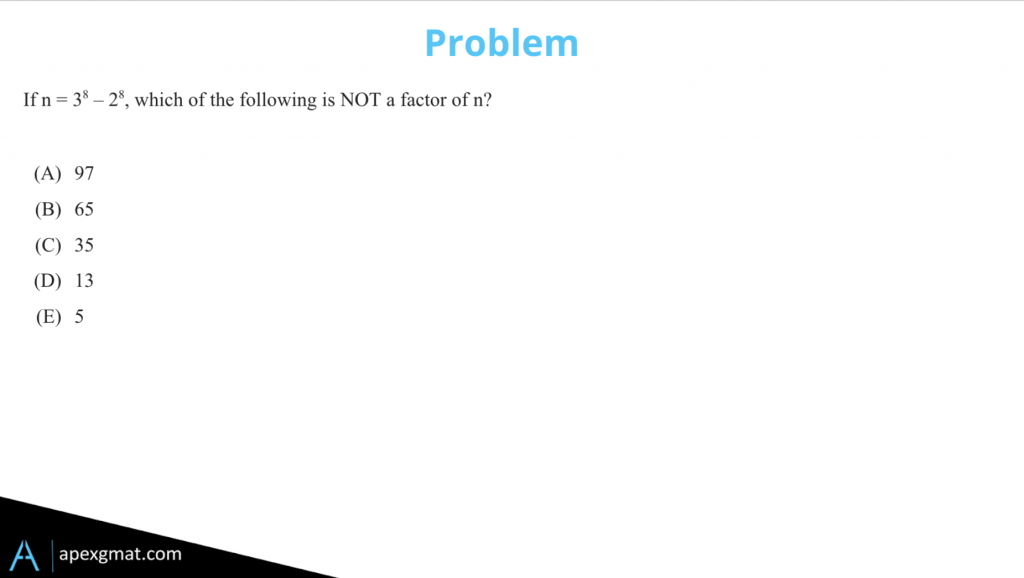Hey guys, check out this problem. This is an example of a problem that requires daisy-chaining together or linking together several key algebraic insights in order to answer it.###### GMAT Prime Factors Problem – Applied Math Solution Path

Notice there’s an applied math solution path. We want prime factors of 3⁸ - 2⁸, and it’s just reasonable enough that we can do the math here. And the GMAT will do this a lot, they’ll give us math that’s time-consuming, but not unreasonably time-consuming in order to just draw us into an applied math solution path. We’ll take a look at this really quickly.

3⁸ is the same as 9⁴.
3⁸ = 3²*⁴= (3²)= 9
9 = (9 * 9)² = 81²
81 * 81 = 6,561

9 * 9 is 81² – about 6,400 or if we want to get exact, which we do need to do here because we’re dealing with factors, 81 * 81 is 6,561. Don’t expect you to know that, it can be done in 20 seconds on a piece of paper or mentally. And then 2⁸, that one you should know, is 256. And then, 6,561 – 256 = 6,305.

So now we need to break down 6,305 into prime factors. You know how to do that using a factor tree, so I’m going to zoom us right into a better solution path because I don’t want to give away the answer.

###### GMAT Prime Factors Problem – Another Solution Path

Notice that 3⁸  and 2⁸ are both perfect squares so we have the opportunity to factor this into (3– 2) * (3 + 2). Once again, the first term is a difference of two squares, the second term we can’t do anything with. So we break down that term, and lo and behold, (3² – 2²) * (3² + 2²) * (3 + 2), and once again we can factor that first term out into (3 + 2), (3 – 2), and so on. We work these out mathematically, and they’re much easier and more accessible mathematically, and we get 3 – 2 = 1 which obviously is a factor of everything. 3 + 2 = 5, 3² + 2² = 9 + 4 = 13, and then 3 + 2⁴ = 81 + 16 = 97.

So now we’ve eliminated everything, except B and C, 65 and 35. This is where the other piece of knowledge comes in. Since we have factors of 5 and 13. 65 must also be a factor because it’s comprised of a 5 and a 13. 35 requires a 7. We don’t have a 7 anywhere, so the correct answer choice is C, 35.

###### GMAT Prime Factors Problem – Takeaways

So the big takeaways here are, that, when provided with some sort of algebraic expression like this, look for a factoring pattern. And, when it comes to prime factorization, remember, that if you break it down into the basic prime factor building blocks, anything that is a product of those building blocks also exists as a factor.

Hope this helped and good luck!

Found it helpful? Try your hand at this GMAT problem, GMAT Prime Factorization (Anatomy of a Problem).